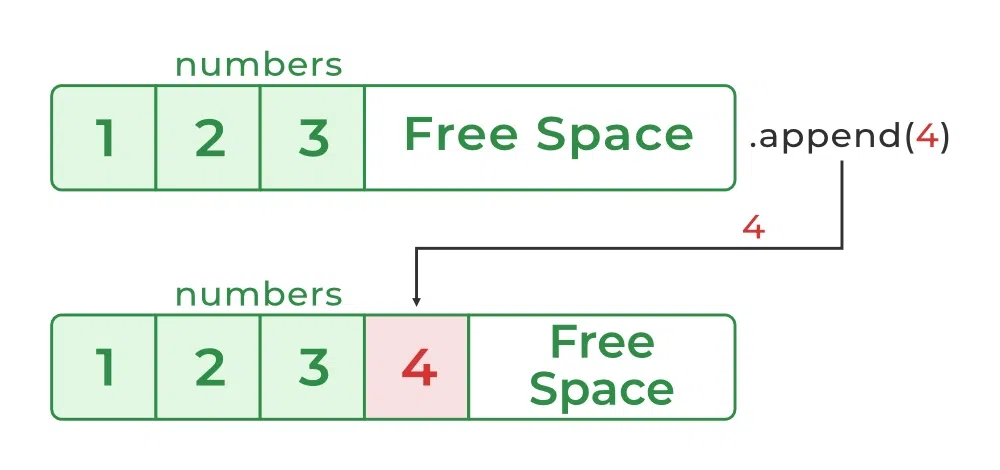Open In App

# Python List append() Method

Lists are fundamental data structure in Python that allows for storing and manipulating a collection of objects. The Python List append() method is used for appending and adding elements to the end of the Python List.

## Syntax of List append()

The syntax of the Python List append() method is as follows:

Syntax: list.append(item)

Parameters:

1. item: an item (number, string, list etc.) to be added at the end of the list, The parameter is mandatory and omitting it can give an error.

Returns: N/A (The method doesn’t return any value)

## How To Add Elements to a List in Python

The list is a sequence datatype used to store elements. Lists are mutable i.e., we can add/remove elements. There are many different methods to add elements to a list in Python.

Here are some ways by which you can add elements to a List are given below.

Adding elements to the end of a list with Python’s append() method increases the list’s size. It offers a practical method to add one or more elements to a list that already exists. Here is an example of using the List Append() method.### Example 1: AddingElement to the List Using Append() Method

In this example, we will append a single element at the end of the given list using the List append() method in Python.

## Python3

 `# my_list``my_list ``=` `[``'geeks'``, ``'for'``]`` ` `# Add 'geeks' to the list``my_list.append(``'geeks'``)`` ` `print``(my_list)`

Output:

```['geeks', 'for', 'geeks']

```

Time complexity: O(1)
Space complexity: O(1)

### Example 2: Adding List to the List

In this example, we will append a complete list at the end of the given list.

## Python3

 `# my_list``my_list ``=` `[``'geeks'``, ``'for'``, ``'geeks'``]`` ` `# another list``another_list ``=` `[``6``, ``0``, ``4``, ``1``]`` ` `# append another_list to my_list``my_list.append(another_list)`` ` `print``(my_list)`

Output:

```['geeks', 'for', 'geeks', [6, 0, 4, 1]]
```

Note: A list is an object. If you append another list onto a list, the parameter list will be a single object at the end of the list. If you want to append the elements of a list to another list you can use Python’s List extend() method.
Time complexity: O(1) or constant time. This is because lists can be accessed randomly using indexing, so the last element can be accessed easily in O(1) time.

### Example 3: Adding Element in List Using Concatenation Operator

Another way to add elements to a list is using the concatenation operator( ‘+’ ), in this we create a new list by combining the existing list with another list that contains the new element.

## Python3

 `# Existing list``my_list ``=` `[``9``, ``5``, ``7``]   ``new_element ``=` `3``my_list ``=` `my_list ``+` `[new_element]``print``(my_list)        `

Output:

```[9, 5, 7, 3]
```

Time complexity: O(1)
Space complexity: O(1)

### Example 4: Adding Element to a List Using List Slicing

Another way to add elements to a list is using list slicing, this allows you to modify a portion of a list by assigning new values to it. To add elements to specific positions within a list, we can use list slicing with the insert() method.

## Python3

 `# Existing list``my_list ``=` `[``3``, ``4``, ``6``]  ``# Insert element 5 at index 2``my_list.insert(``2``, ``5``) ``print``(my_list)`

Output:

```[3, 4, 5, 6]

```

Time complexity: O(1)
Space complexity: O(1)

Note: If you need to add items of a list (rather than the list itself) to another list, use the extend() method.